# Bayesian LSTMs in Medicine

This paper gives a demonstration of using Bayesian LSTMs for classification of medical time series, which can improve the accuracy compared with standard LSTMs.

This paper gives a demonstration of using Bayesian LSTMs for classification of medical time series, which can improve the accuracy compared with standard LSTMs. First, I would like to give you a short introduction of RNNs (Recurrent Neural Networks), because LSTMs (Long Short-Term Memory Networks) is a specific type of RNN. For humans, our thought doesn’t always start from scratch. For example, when you read an article, the preceding text (that you understand) can help you understand the text you are currently reading. But for some traditional neural networks, they just abandon the previous content and start from scratch. What they represent is that your memory is persistent, but the traditional neural network is not. RNNs can solve this problem theoretically, but the truth is that due to its simple structure (which usually has only one tanh layer), it cannot handle long-term dependencies. LSTMs is proposed with a more complicated structure, which has four neural network layers, to make it possible to solve the problem of long-term dependencies.

### Introduction

In medicine, doctors need to evaluate multiple parameters, and based on a complex mixture of assumptions and intuitions, make their decisions. RNNs have been proved to achieve some of the best results in reference [1, 2, 3, 4]. But due to the fact that RNNs’ models cannot provide a certain measure of the practitioners’ decisions, the effect of treatment for a given patient cannot be deterministic. Bayesian probability theory can help to reason a model’s uncertainty in a mathematical way. There are two key benefits by using the Bayesian deep learning, one is to increase the classification accuracy of medical signals, and the other is to provide a measure of confidence in the model decisions. The authors also point out that even if the conventional Bayesian approaches introduce too much overhead, it can be implemented into the online classification in a clinical setting, which can help save the overall cost.

### Intuition behind the Methods

Bayesian LSTMs is a kind of LSTM that uses dropout to perform Bayesian inference. It uses the simple one, which consists of three gates (input, output, forget) and a cell unit. The gate uses a sigmoid activation function, while input and cell state usually use tanh to convert.

### Methods

In this paper, the authors’ implementation of LSTM is based on reference  using Tensorflow . There are 4 gates in each cell of the LSTM: input i, output o, forget f, and input modulation gates g. The functions are shown below:The internal state ct is to represent as cell, and it is updated additively. σ represent the non-linear sigmoid activation. W∗ and U∗ are the input and hidden weight matrices, respectively, with biases b∗. After having these, we can compute the input to each gate’s non-linearity by using the following single matrix multiplication: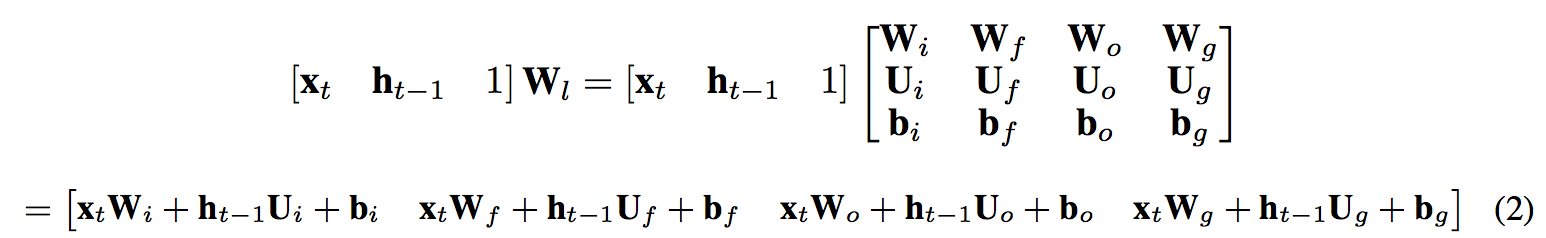And according to reference , this approach to generate the matrix is a faster forward-pass.

### Bayesian LSTM

In this part, the authors give a strict derivation process of Bayesian LSTM. I’ll show you the key stages, and if you are interested in more details, it can be found in the paper.

Dropout method is leveraged to perform Bayesian inference with LSTMs. Given the observed labels Y and data X as in Equation 3.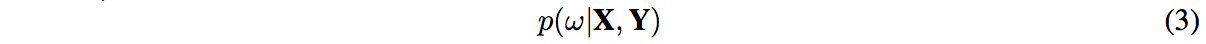The authors in this paper use variational inference to make an approximation of posterior distribution because it cannot be tracked in a general way. They minimize the reverse Kullback Leibler (KL) divergence between this approximate distribution and the full posterior to learn about the network’s weights. In Equation 4, q(ω) is a distribution on those matrices whose columns are randomly set to zero.For LSTM, each matrix Wl has dimensions Kl−1 × Kl. q(ω) can be defined as:The authors re-write the operation (omitting biases for brevity) by LSTM’s definitions in Function 1:The output can be defined as fy(hT ) = hT Wy + by. To emphasize the dependence on ω, the functions are written as fhω and fyω . To approximate the posterior distribution q(ω), the authors give the function:This can be approximated with MC integration:Then the minimization objective can become as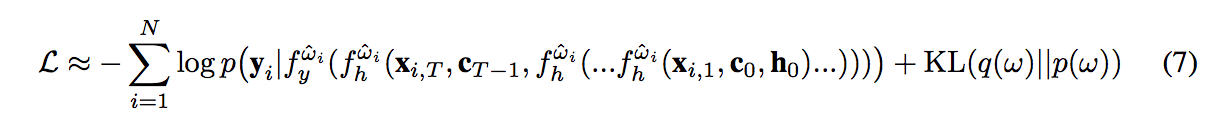For each layer l every weight matrix column wlk the approximating distribution is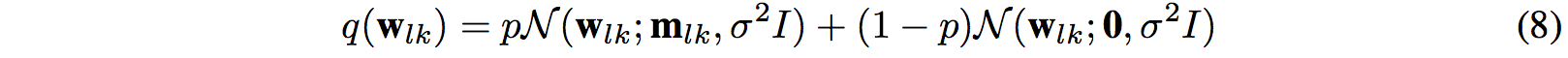The KL term in Equation 7 can be approximated asPredictions can be approximated by the standard forward pass for LSTMs like propagating the mean of each layer to the next (standard dropout approximations), or approximating the posterior with q(ω) for a new input x∗ as shown in the following function:With the use of naive dropout, the minimization objective can be Function 9:The difference between variational and naive dropout approaches can be seen in Figure 1. Here the distributions are the hidden outputs in Equation 6, and it is plotted over 150 epochs for a model trained on MNIST dataset that I will describe later. It shows the percentiles of the hidden layer outputs, which are overall time steps for the same arbitrary input sample for each epoch. Table 1 shows that both approaches have similar performance. But according to the authors’ hypothesis, the naive approach has a narrow distribution on the first layer, and in the second layer the variational approach has a concurrent narrow band of exploration. It was found that in the training simulations for the naive approach, the distributions would vary between different training simulations. But for the variational approach, it is the same for any training simulation.### Experimental implementation

To demonstrate the efficacy of Bayesian LSTMs, the authors use 5 datasets. For each dataset, they use the same LSTM model with a different architecture. A validation set was used, and Dropout was used on only the input and output LSTM connections. Optimization was performed with Adam , and the learning rate is 0.01, the minibatch size is 256. Here the authors provide the way how they configure the parameters for each dataset.

1). MNIST
it was processed in scanline order , There are 2 hidden layers with 128 units in each layer.

2). MIT-BIH arrhythmia dataset
It contains 48 half-hour excerpts of electrocardiogram (ECG) recordings from 47 patients. Its percentage of train:test:validation is 50:40:10. The model is a single hideen layer of 128 units and the dropout probability is 0.3.

3) Physionet/Compute in cardiology challenge 2016
There is 4,430 phonocardiogram (PCG) recordings in this dataset, and 3,126 were used for training. 301 validation samples were extracted from the training dataset. The data were provided with normal and abnormal heartbeats. The model has 2 hidden layers of 128 units, and the dropout rate is 0.25. The model will return a score that was evaluated by means of online submission.

4) Neonatal intensive care unit dataset
It contains the first 48 hours of vital signs for 3 neonatal intensive care unit (NICU) patients. The signals contained ECG, blood pressure and oxygen saturation. The model has a single hidden layer of 64 units and the dropout rate is 0.1. The random split of 50:40:10 was used.

5) Traumatic brain injury dataset
The data was from traumatic brain injury (TBI) patients. The dataset contains 19 variables recorded for 101 patients, of which 34 were females, and the age ranged from 15 to 76. The model has a single hidden layer of 128 units and the dropout rate is 0.4. A random split of 50:40:10 was used.

### Results

Table 1 shows the results from the datasets analyzed. The values are the average rate for 10 times running. We can see in the table that Bayesian LSTM used for classification of medical time series provides an improvement.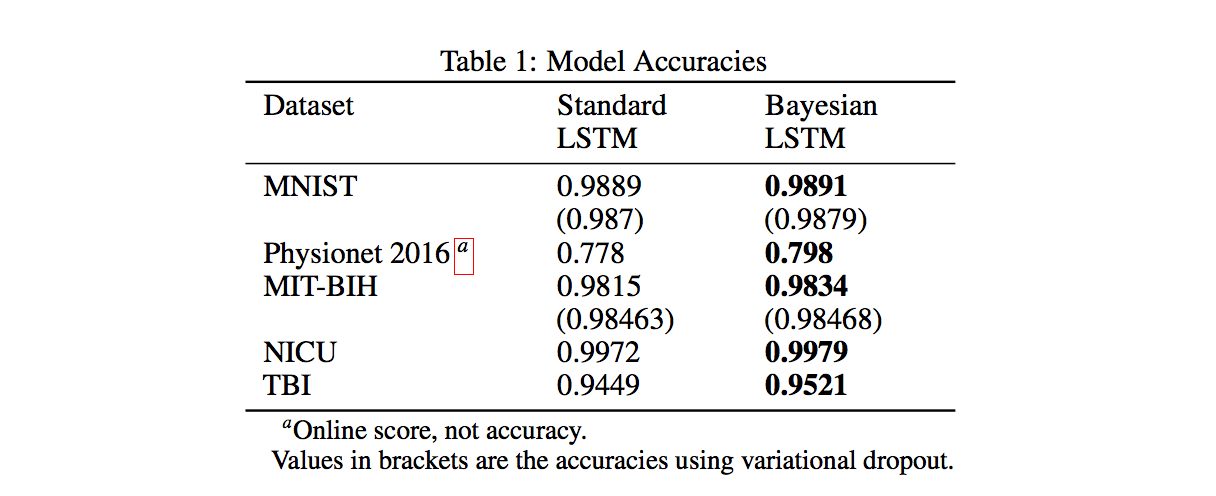In Figure 2, the authors juxtapose confident and uncertain Bayesian LSTM classified medical signals. It should be mentioned that only estimated class is produced as output in standard LSTMs. The figure shows that when the signals look noisy or abnormal, the model is uncertain. When this condition occurs, the practitioners should do further investigation.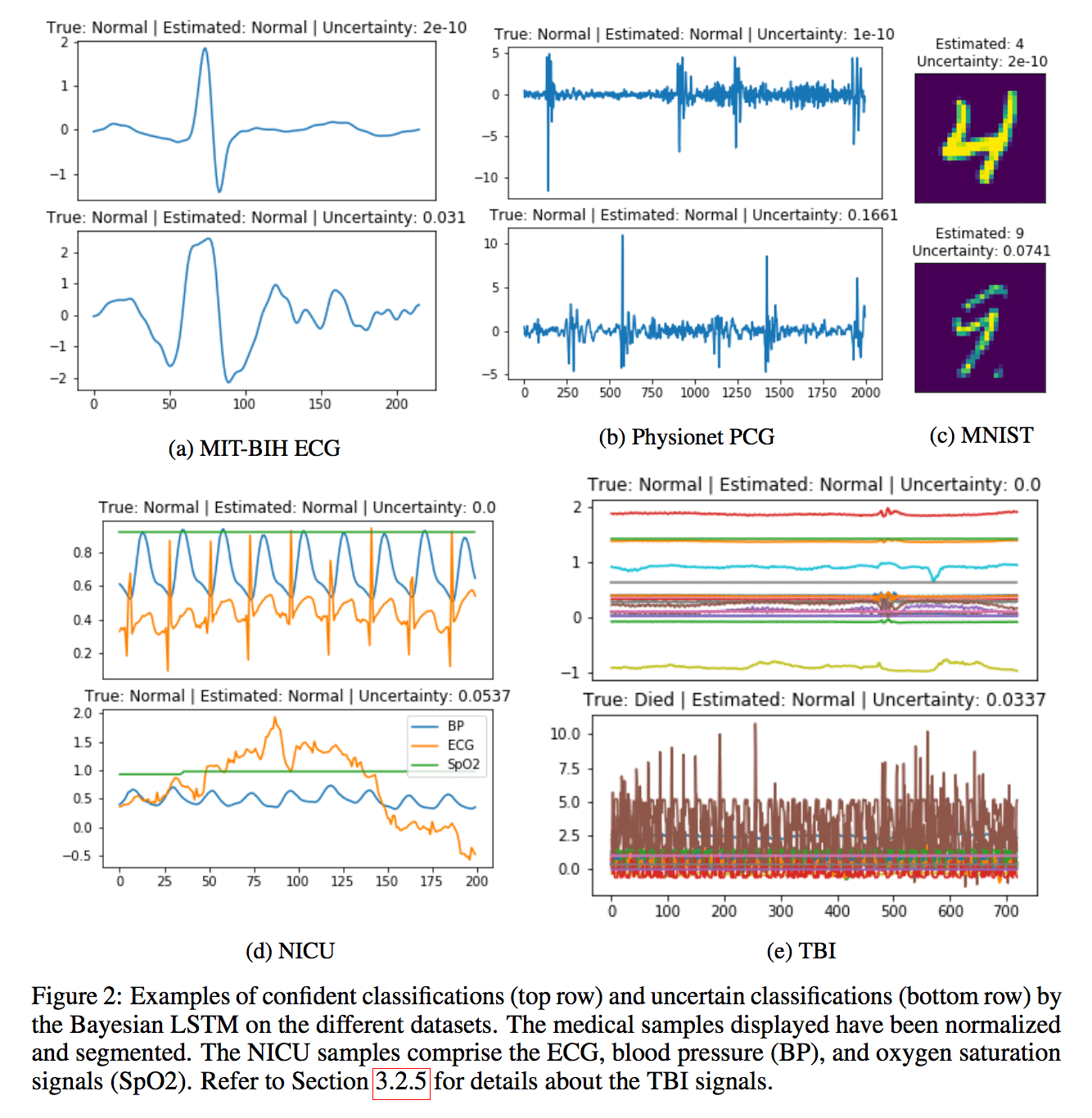### Discussion

Here the authors give us mainly three points:

1. We find the improvement of the Bayesian compared with the traditional is not that high, and the authors give the reason. According to reference , the LSTMs will have poor performance on signals longer than 1000 time steps. But if the authors split medical signals like this, this will confuse the model during training.
2. The authors also give the recommended dropout rate, which is to keep it lower than 0.2.
3. Due to the samples being independent, MC dropout is a highly parallelized method even if it is computationally expensive.

### Conclusion

It shows that the conventional deep learning technique for time series have the following two advantages: (i) It helps perform the quantifying model decisions by providing a vital additional output. (ii) It can improve the model accuracy. Additionally, in medical machine learning, the methods for quantifying aleatoric uncertainty can also give valuable benefits.

### Reference

 Lipton, Zachary C., et al. “Learning to diagnose with LSTM recurrent neural networks.” arXiv preprint arXiv:1511.03677 (2015).
 Choi, Edward, et al. “Doctor ai: Predicting clinical events via recurrent neural networks.” Machine Learning for Healthcare Conference. 2016.
 Jagannatha, Abhyuday N., and Hong Yu. “Bidirectional rnn for medical event detection in electronic health records.” Proceedings of the conference. Association for Computational Linguistics. North American Chapter. Meeting. Vol. 2016. NIH Public Access, 2016.
 Harutyunyan, Hrayr, et al. “Multitask Learning and Benchmarking with Clinical Time Series Data.” arXiv preprint arXiv:1703.07771 (2017).
 Hochreiter, Sepp, and Jürgen Schmidhuber. “Long short-term memory.” Neural computation 9.8 (1997): 1735-1780.
 Abadi, Martín, et al. “Tensorflow: Large-scale machine learning on heterogeneous distributed systems.” arXiv preprint arXiv:1603.04467 (2016).
 Gal, Yarin. Uncertainty in deep learning. Diss. PhD thesis, University of Cambridge, 2016.
 Kingma, Diederik, and Jimmy Ba. “Adam: A method for stochastic optimization.” arXiv preprint arXiv:1412.6980 (2014).
 Cooijmans, Tim, et al. “Recurrent batch normalization.” arXiv preprint arXiv:1603.09025 (2016).
 Neil, Daniel, Michael Pfeiffer, and Shih-Chii Liu. “Phased LSTM: Accelerating recurrent network training for long or event-based sequences.” Advances in Neural Information Processing Systems. 2016.

Author: Shixin Gu | Resource: https://arxiv.org/abs/1706.01242 | Reviewer: Hao Wang